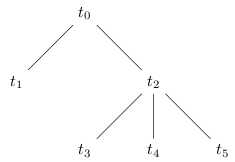# Trees

A directed graph is a pair `(T,R)` where `T` is a set and `R` is a binary relation on `T`, i.e., `R⊆T×T`.

The finite sequence `t0`, `t1`, ..., `tn` where `ti∈T` and `tiRti+1`, is called a path.

An infinite sequence `t0`, `t1`, ..., is a path if every finite subsequence starting with `t0` is a path in the previously defined sense.

A tree is a triple `(T,R,r)` such that `(T,R)` is a directed graph, `r∈T` and for all `t∈T` there is a unique path from `r` to `t`. The elements of `T` are called the nodes of the tree. The node `r` is called the root of the tree. The elements of `R` are called the edges, or arcs, of the tree. For an edge `(t,s)∈R`, `t` is called the parent node, and `s` is called the child node. A node `t` is called a leaf if it has no children.

A branch is a path that either terminates at a leaf, or is infinite. In a finitely branching tree, there is no node which has an infinite number of children.

## Example

First we define the set of nodes and edges:

```	```
T={t0,t1,t2,t3,t4,t5}
R={(t0,t1), (t0,t2),
(t2,t3), (t2,t4), (t2,t5)}
```
```

The diagram below shows the tree `(T,R,t0)`.## References

Doets, Kees. From Logic to Logic Programming. MIT Press, 1994.